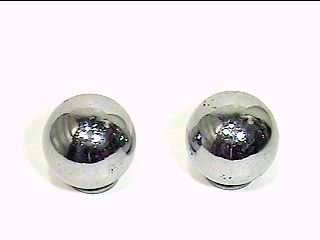# Two Balls !

Algebra Level 1Given that the mass of ball A is four times the mass of ball B. The product of the mass of the two balls is 256(kg^2). Find the total mass of two balls.

P/S : it is kg square = kg to the power of 2

For example : 5kg x 6kg = 30(kg^2)

Sorry for the confusing mistake.

×